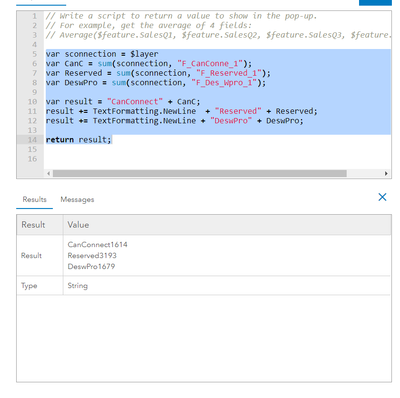# Field totals for entire layer

841
8
05-10-2021 01:22 PM
Labels (1)New Contributor III

Hello folks,

can someone put me in the right direction or provide an example for me of the following arcade statement?

I would like to display the  the sum of a field for the an entire  layer not just the selection. I would like to show the totals of three separate fields in an arcgis online popup

Example:

Field 1 Total (of all records)

Field 2 Total (of all records)

Field 3 Total (of all records)

any help will be appreciated

Thanks!

2 Solutions

Accepted SolutionsNew Contributor III

figured it out- Thanks again

AaronbyEsri Esteemed Contributor

Hi @aroininen ,

It is possible to apply a filter on the data. Have a look at the expression below. We define a SQL query (make sure you use a valid query and the correct field names). We use a Filter to apply the query and provide the filtered featureset to the sum functions.

``````// make sure you use the extact field name
var sql = "ServiceArea IN (1,4,5,8,9)";

// filter the layer with the query
var fs = Filter(\$layer, sql);

// get the totals
var Canc = Sum(fs, "F_CanConne_1");
var Reserved = Sum(fs, "F_Reserved_1");
var DeswPro = Sum(fs, "F_DesWpro_1");
var Total = Canc + Reserved + DeswPro;

// structure the result
var result = "CanConnect: " + CanC;
result += TextFormatting.NewLine + "Reserved: " + Reserved;
result += TextFormatting.NewLine + "DeswPro: " + Deswpro;
result += TextFormatting.NewLine + "Total: " + Total;

// return the result
return result;``````

8 RepliesbyEsri Esteemed Contributor

Hi @aroininen ,

The first question would be to know if the feature you click on is part of the layer where you want to get the totals from (this would allow us to use \$layer)? If not, is the layer in the map (you would probably use FeatureSetByName? If that is not the case, the way to access the layer will be a bit different using FeatureSetByPortalItem.

The second question is,  do you want to return 3 separate values and handle them as separate virtual fields (which would need an Arcade expression for each total) or is returning a text with the 3 totals what you are looking for?

Have a look at the example below:

``````// access the layer of the feature the user clicked on
var fs1 = \$layer;

// access another layer that is available in the map
var fs2 = FeatureSetByName(\$map,"Parcels_And_Buildings - Tax Parcels")

// calculate the sum of floor count of the first featureset
var tot1 = Sum(fs1, "FLOORCOUNT");

// calculate the sum of the Residential Floor Area fom the seconf featureset
var tot2 = Sum(fs2, "RESFLRAREA");

// calculate the sum of the Building Area fom the seconf featureset
var tot3 = Sum(fs2, "BLDGAREA");

// create a string and append each total on a new line
var result =  "Floorcount: " + tot1;
result += TextFormatting.NewLine + "Residential Floor Area: " + Round(tot2, 0);
result += TextFormatting.NewLine + "Building Area: " + Text(tot3, "000.000.000 m²");

// return the formatted text
return result;``````

This will return a single text containing the 3 sums:

``````Floorcount: 26405
Residential Floor Area: 133370425
Building Area: 397.900.000 m²``````New Contributor III

got it!! thanks!!

yes - using same layer as clicked and do not require a separate field.

is it possible to add a line to add up the total of the three?

Thank you so much!New Contributor III

figured it out- Thanks again

AaronNew Contributor III

OK!

the results are for the whole dataset -  I thought it would reflect the filter that is on the data..

Can I filter within the expression

i.e service area IN (1,4,5,8,9) ....?

Thoughts

AaronbyEsri Esteemed Contributor

Hi @aroininen ,

It is possible to apply a filter on the data. Have a look at the expression below. We define a SQL query (make sure you use a valid query and the correct field names). We use a Filter to apply the query and provide the filtered featureset to the sum functions.

``````// make sure you use the extact field name
var sql = "ServiceArea IN (1,4,5,8,9)";

// filter the layer with the query
var fs = Filter(\$layer, sql);

// get the totals
var Canc = Sum(fs, "F_CanConne_1");
var Reserved = Sum(fs, "F_Reserved_1");
var DeswPro = Sum(fs, "F_DesWpro_1");
var Total = Canc + Reserved + DeswPro;

// structure the result
var result = "CanConnect: " + CanC;
result += TextFormatting.NewLine + "Reserved: " + Reserved;
result += TextFormatting.NewLine + "DeswPro: " + Deswpro;
result += TextFormatting.NewLine + "Total: " + Total;

// return the result
return result;``````New Contributor III

It doesn't seam to use the filter...? did I miss something...?

var sql = "ServiceAre_1 IN (2,5,6,8,9)";
var fs = Filter (\$layer,sql);

var sconnection = \$layer
var CanC = sum(sconnection, "F_CanConne_1");
var Reserved = sum(sconnection, "F_Reserved_1");
var DeswPro = sum(sconnection, "F_Des_Wpro_1");
var Total = Canc + Reserved + DeswPro;

var result = "CanConnect: " + CanC;
result += TextFormatting.NewLine + "Reserved: " + Reserved;
result += TextFormatting.NewLine + "DeswPro: " + DeswPro;
result += TextFormatting.NewLine + "Total: " + Total;

return result;

CanConnect: 1614

Reserved: 3193

DeswPro: 1679

Total: 6486byEsri Esteemed Contributor

Hi @aroininen ,

If you look at my code you will see that the Sum is using "fs" (the filtered featureset) and not "sconnection" (the \$layer). This is why it is not working for you.New Contributor III

awe yes.. thankyou!